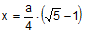# Problem 524: Circle, Equilateral Triangles, Midpoint, Side, Measurement. Level: High School, SAT Prep, College geometry

 The figure shows an equilateral triangle ABC (side a) inscribed in a circle C1. The point D is the midpoint of BC and E and F are on the circle C1. If the triangle DEF is equilateral (side x), prove that. Post a comment or solution.Reference: Fukagawa Hidetoshi, Tony Rothman, "Sacred Mathematics: Japanese Temple Geometry" (Princenton University Press, 2008). See also: Problem 525. Circles, Diameter, Tangent, Radius, Congruence, Measurement. Problem 523. Tangent Circles, Diameter Perpendicular, Collinearity. Problem 522. Right Triangle, Circle, Diameter, Tangent.
 Home | Search | Geometry | Problems | All Problems | Sangaku | 521-530 | Email | By Antonio Gutierrez